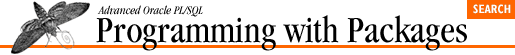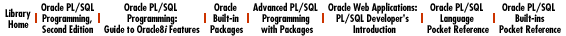home | O'Reilly's CD bookshelfs | FreeBSD | Linux | Cisco | Cisco Exam

### 5.3 PLV: Top-Level Constants and Functions

The PLV (PL/Vision) package provides a single collection point for constants and basic functions used throughout the PL/Vision library of packages. See Chapter 6, PLV: Top-Level Constants and Functions for details.

### 5.3.1 PL/Vision constants

``` dbtab CONSTANT VARCHAR2(2) := 'DB';```
``` pstab CONSTANT VARCHAR2(2) := 'PS';```
``` file CONSTANT VARCHAR2(2) := 'F';```
``` string CONSTANT VARCHAR2(2) := 'S';```
``` stdout CONSTANT VARCHAR2(2) := 'SO';```

Names of different repositories supported within PL/Vision. These are mostly used by PLVio and by users of PLVio to set up the source and target repositories for PL/SQL code.

``` c_datemask CONSTANT VARCHAR2(100) :=```
``` 'FMMonth DD, YYYY FMHH24:MI:SS'```

The default date format mask for PL/Vision .

### 5.3.2 Anchoring datatypes

``` plsql_identifier VARCHAR2(100) := 'IRRELEVANT';```
``` max_varchar2 VARCHAR2(32767) := 'IRRELEVANT';```
``` vcmax_len CONSTANT INTEGER := 32767;```

The plsql_identifier variable offers a standard format for the declaration of any variables that will hold PL/SQL identifiers, such as table and column names.

The max_varchar2 variable offers a standard format for the declaration of any variables that require the maximum possible size for VARCHAR2 variables, which is 32,767 bytes and also reflected by the value of the vcmax_len constant.

### 5.3.3 Setting the date format mask

``` PROCEDURE set_datemask (datemask_in IN VARCHAR2 := c_datemask);```

Sets the string used as the default date format mask within PL/Vision.

``` FUNCTION datemask RETURN VARCHAR2;```

Returns the string used as the default date format mask within PL/Vision.

### 5.3.4 Setting the NULL substitution value

``` PROCEDURE set_nullval (nullval_in IN VARCHAR2);```

Sets the string used as the substitution value for NULLs within PL/Vision.

``` FUNCTION nullval RETURN VARCHAR2;```

Returns the current NULL substitution value.

### 5.3.5 Assertion routines

``` assertion_failure EXCEPTION;```

Exception raised by the various assertion routines when the assertion fails.

``` PROCEDURE assert (bool_in IN BOOLEAN, stg_in IN VARCHAR2 := NULL);```

Does nothing if the Boolean argument evaluates to TRUE. Otherwise (for FALSE or NULL), it raises the assertion_failure exception and displays the message.

This same behavior holds for the other assertion routines shown below.

``` PROCEDURE assert_notnull```
``` (val_in IN VARCHAR2, stg_in IN VARCHAR2 := NULL);```
``` PROCEDURE assert_notnull```
``` (val_in IN DATE, stg_in IN VARCHAR2 := NULL);```
``` PROCEDURE assert_notnull```
``` (val_in IN NUMBER, stg_in IN VARCHAR2 := NULL);```
``` PROCEDURE assert_notnull```
``` (val_in IN BOOLEAN, stg_in IN VARCHAR2 := NULL);```

``` PROCEDURE assert_inrange```
``` (val_in IN DATE, ```
``` start_in IN DATE := SYSDATE, end_in IN DATE := SYSDATE+1,```
``` stg_in IN VARCHAR2 := NULL, truncate_in IN BOOLEAN := TRUE);```
``` PROCEDURE assert_inrange```
``` (val_in IN NUMBER, start_in IN NUMBER, end_in IN NUMBER, ```
``` stg_in IN VARCHAR2 := NULL);```

### 5.3.6 Miscellaneous programs

``` FUNCTION boolstg (bool_in IN BOOLEAN, stg_in IN VARCHAR2 := NULL)```
``` RETURN VARCHAR2;```

Returns a string representing the value of the Boolean argument: TRUE if the Boolean argument is TRUE, FALSE if FALSE, and NULL if NULL.

``` FUNCTION errm (code_in IN INTEGER := SQLCODE) RETURN VARCHAR2;```

Returns the error message provided by SQLERRM. Encapsulation inside this function allows SQLERRM to be referenced inside a SQL statement.

``` FUNCTION now RETURN VARCHAR2;```

Returns the current date and time using the current PL/Vision date format mask.

``` PROCEDURE pause (secs_in IN INTEGER);```

Pauses your PL/SQL program for the specified number of seconds.5.2 p: a DBMS_OUTPUT Substitute5.4 PLVcase: PL/SQL Code Conversion